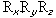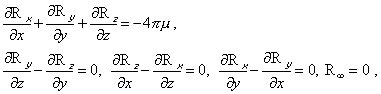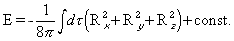### Home | Up One Level | Previous | Next

WALTER RITZ

#### Annales 179 (Oeuvres 345)

If we consider the electromagnetic theories in their present form as a general basis for the explanation of physical phenomena, a role that only Mechanics played until now, it will suffice, at first, to ask ourselves if we can place gravitation in this general scheme.  Is the notion of field, (Oeuvres 346) with its consequences, capable of being applied? The response given by Maxwell1 is negative.

In introducing the forcewhich gravitation exercises at a point xyz in space on a unit of mass, we can well, as in electrostatics, determine this force by the system of equations (µ = density)And the value of the energy will be(the unit mass being chosen properly).  Since there is attraction, the integral has the sign (-). But, says Maxwell

the energy being essentially positive, for E to be positive, it will be necessary to choose an enormous value for the constant, greater that the greatest value that the integral could attain for all possible positions of the bodies. The intrinsic energy of the gravitational field is constrained to decrease wherever gravity is sensible. “Since it is impossible for me to understand how a medium could possess such properties, I cannot pursue research, in this vein, into the cause of gravitation."

We can again say that the condition of stability of a continuous medium, elastic or otherwise, is always such that the energy is minimum when the deformation is zero; here; it is maximum for R = 0. The gravitational field would be in unstable equilibrium at infinity and wherever R is zero.

The notion of field doesn’t seem applicable to gravitation; it shouldn’t therefore be an issue to consider as a general base for the explanation of physical phenomena.

On the contrary, the law of elementary action, which results from Lorentz’s theory, if we replace the electric charges by (Oeuvres 347) masses can, as in the similar laws of Weber, Gauss, etc., replace the classical law of gravitation, without the new terms and the propagation they introduce having an appreciable influence on astronomical phenomena.2  These terms are in fact second order3 and therefore extremely small.  We know that Laplace had arrived at the

### Home | Up One Level | Previous | Next

#### Top

1 MAXWELL, Scientific Papers, volume 1, p. 570.

2 LORENTZ, Zittings verslay, Amsterdam, 8, 1900, p. 603.  – WILKENS, Physic. Zeitschr., 7, 1906, p. 300.

3 This is to say that the factor 1/c = 10exp-10 / 3 is of the second order.